# How do you solve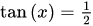?Qian

Solution:

Step:1 Trigonometry ratio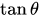is defined as the ratio for perpendicular to the base of right angled triangle with respect to the angle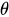.

Consider the right triangle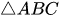such that right angled at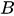as shown in Figure 1.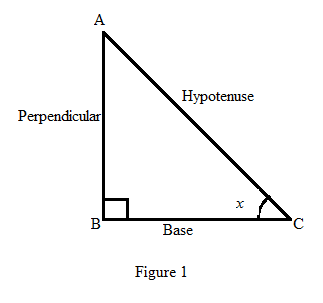Step:2 As it is given that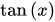is equal to the fraction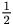. Compare the fraction with corresponding side ratio of perpendicular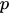and base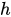.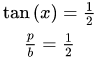From the above comparison, it can be assumed that side perpendicular of the triangle is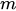and base is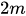.

Use the Pythagoras theorem to calculate the third side (hypotenuse) of the right triangle whose perpendicular isand base is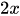.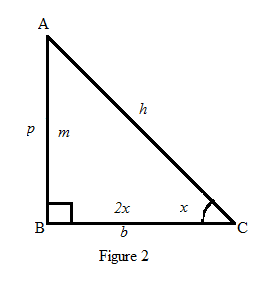Step:3 Pythagoras theorem states that square of hypotenuse is equal to the sum of square of the perbendicular and the square of the base.Further, solve the above equation as follws: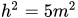Take the square root on both sides and obtain the length of hypotenuseas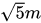.

Step:4  Therefore, the equation ofis equivalent to the equation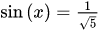or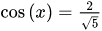for the first quadran or in a angle from 0 to 90 degree.

Step:5 Now, to find the value of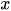in the equationis equivalent to find the value ofin the equation.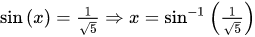The function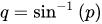or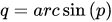is the inverse function of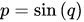Now use the calculator to find the value of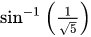as shown below: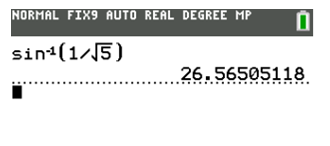Thus, the value foris 26.56505118 degree.

Step:6 Simillarly, the equationis equaivalent to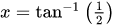and use calculator to obtain the value of x.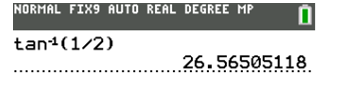Therefore, the principle solution of the equationis 26.56505118 degree approximately.

The principle solution of the equationis 26.56505118 approximately.

Similar Problems:

Problem 1:

How do you solve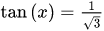?

Solution:

Step:1 Consider the equation.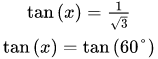Step:2 Compare both sides and obtain the value of x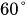.

Principal value ofis.

The solution of the equationin principal region is.

Problem 2:

How do you solve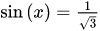?

Solution:

Step:1 Consider the equation.

Step:2 The equationis equaivalent to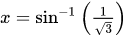and use calculator to obtain the value of x.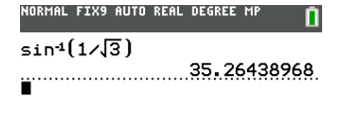Step:3 Therefore, the principle solution of the equationis 35.26438968 degree approximately.

The solution of the equationin principal region is 35.26438968 degree approximately.

### Need homework help now?

tutors online

Related Questions
##### (t) in °C of the pot of soup at time t (in The initial temperature of a pot of soup is 80°C. The temperature minutes) satisfies the differential equation dT di T-20 10 (a) Solve the differential equation, and find an explicit expression for the function (). By separating variables, integrating and manipulating arbitrary constants, . We are also told that so that and so . ...
Notes
Me

OR

Don't have an account?

Join OneClass

Access over 10 million pages of study
documents for 1.3 million courses.

Join to view

OR

By registering, I agree to the Terms and Privacy Policies
Just a few more details

So we can recommend you notes for your school.